A+   a-

# The Rules of DifferentiationNOTE: This lesson is also available as executable worksheets on CoCalc. First, create an account and a project. Then you can copy these files to your project and start working right away. 10-diffrules.ipynb (Jupyter Notebook) and 10-diffrules.sagews (SageMath Worksheet).

### Constant Rule

If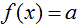and a is a real number, then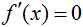.

### Constant Multiple Rule

If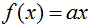and a is a real number, then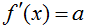.

### Power Rule

Ifand n is a real number, then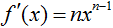.

### Product Rule

If f and g are differentiable at x, then### Quotient Rule

If f is the quotient g(x)/h(x) and h(x) ≠ 0, then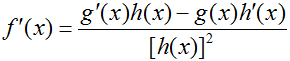### Chain Rule

If g is differentiable at x and f is differentiable at g(x), then